Roman Numerals 3
Set 3 (10 Questions)
Students learn the value of the various Roman Numerals. Students compare the value of Roman and standard numerals. Students have access to links about the historical use of Roman Numerals. This set has slightly larger values than sets 1 & 2.
From Mr. Anker Tests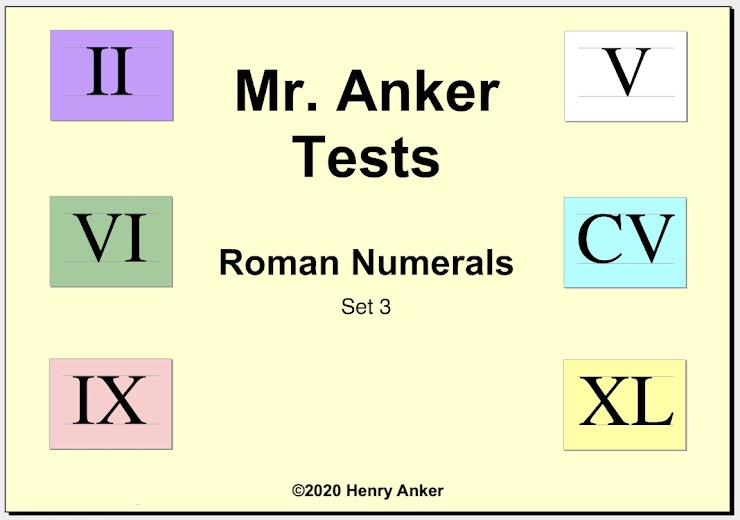Roman Numerals Help Slide 1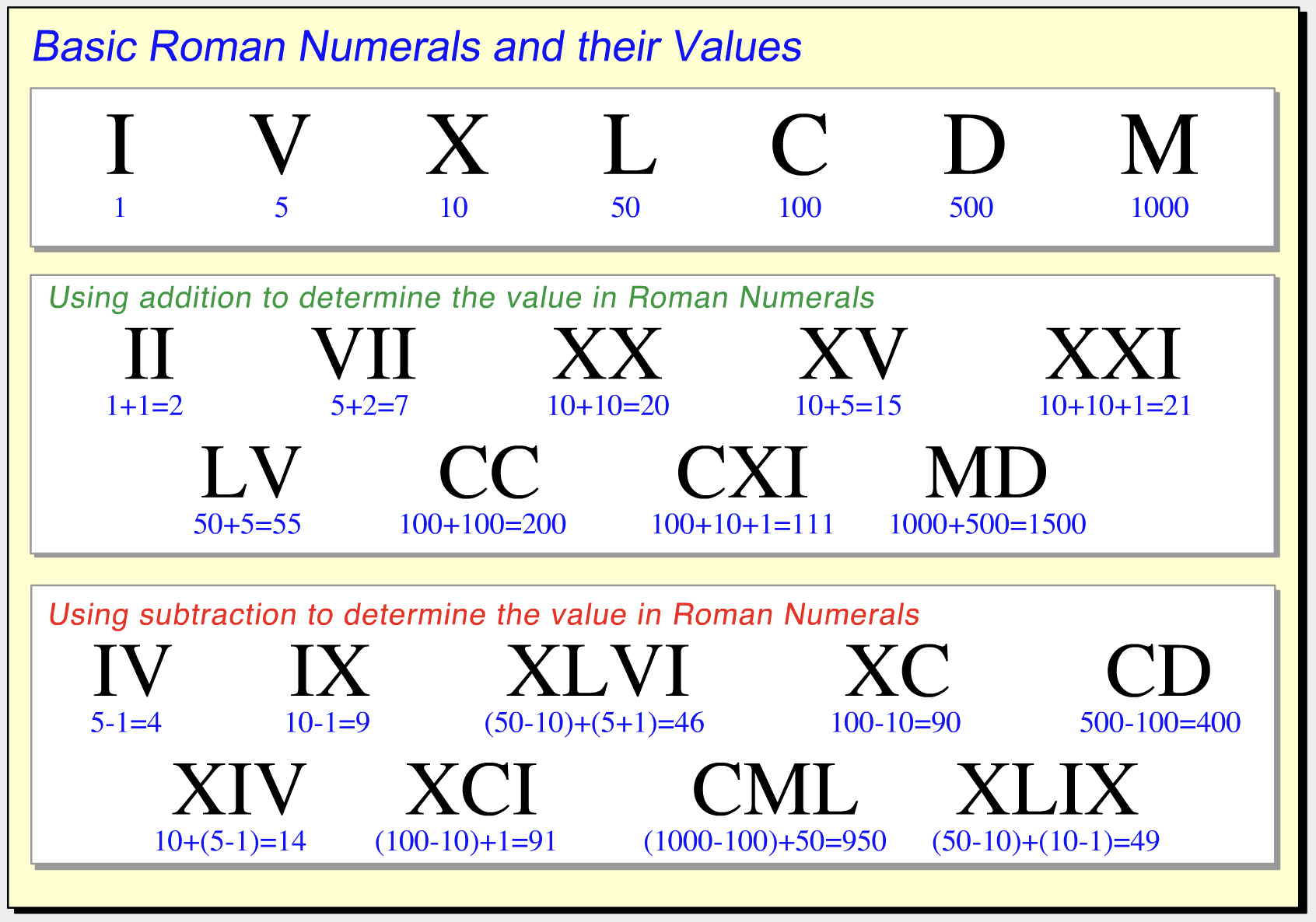This outstanding video can be found on Socratica Math
Roman Numerals Help Slide 2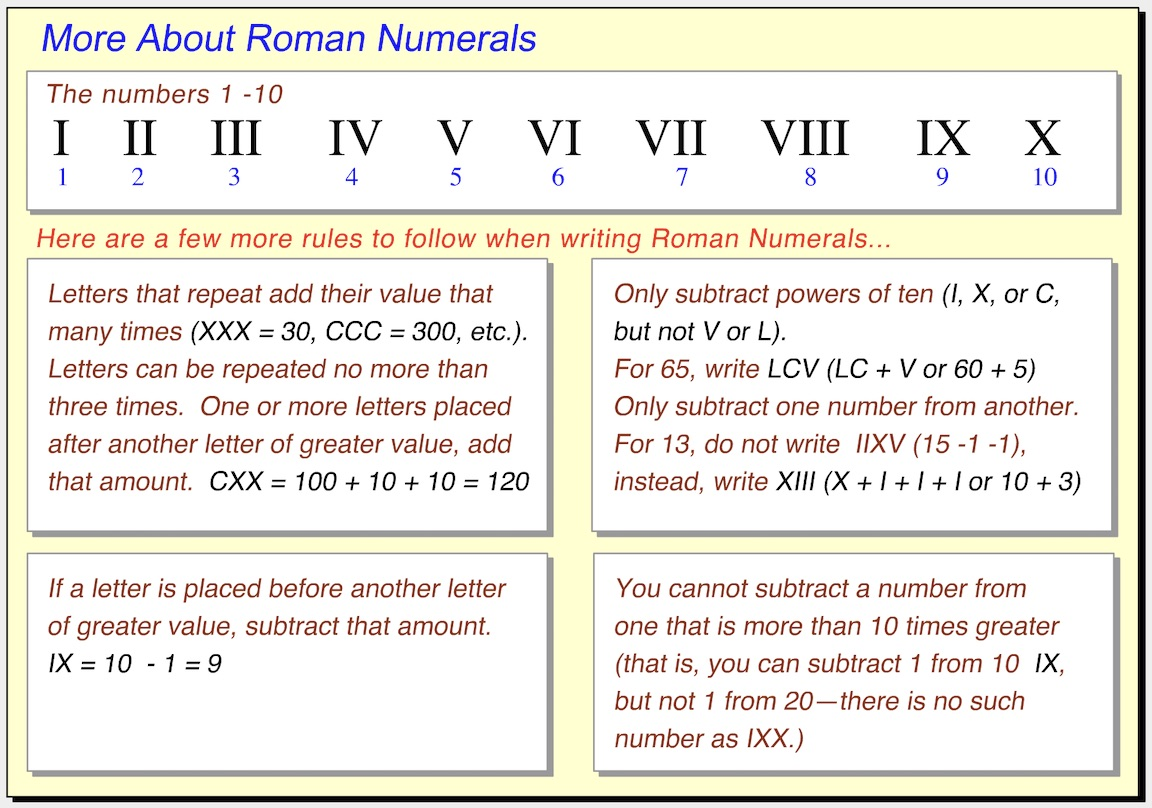1.  The standard numeral 12 is ____________ the Roman Numeral XI. *
1 point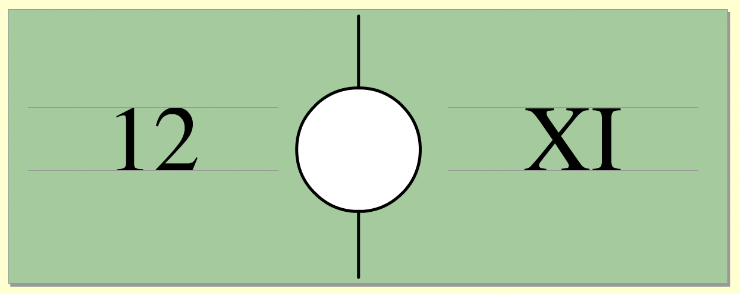2.  Match the standard numeral to this Roman Numeral. *
1 point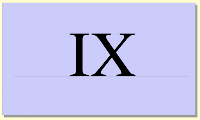3.  The Roman Numeral XV is ____________  the standard numeral 13. *
1 point4.  Match the standard numeral to this Roman Numeral. *
1 point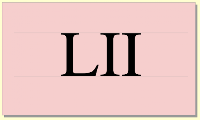5.  The standard numeral 27 is ____________ the Roman Numeral XXVII. *
1 point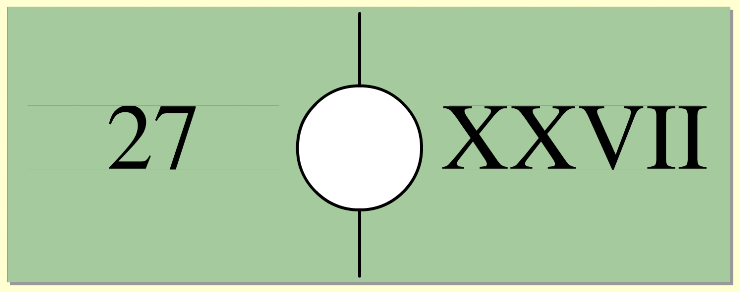6.  Match the Roman Numeral to this standard numeral. *
1 point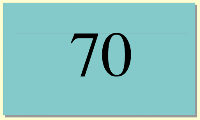7.  The standard numeral 47 is ____________ the Roman Numeral LVII. *
1 point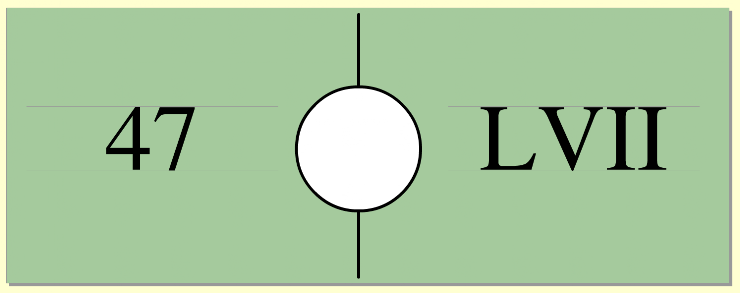8.  Match the Roman Numeral to this standard numeral. *
1 point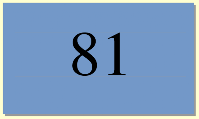9.  Enter, by typing, the standard numeral for this Roman Numeral. *
1 point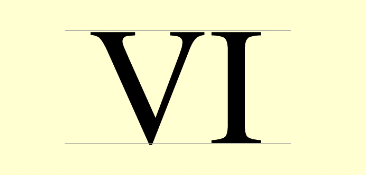10.  Enter, by typing, the standard numeral for this Roman Numeral. *
1 point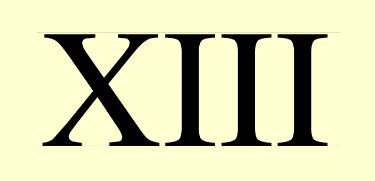Submit
Clear form